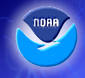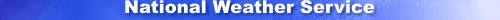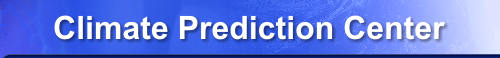home Site Map News Organization Search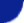Search the CPC About Us    Our Mission    Who We Are Contact Us    CPC Information    CPC Web TeamHOME > Stratosphere Home > Stratosphere-Troposphere Monitoring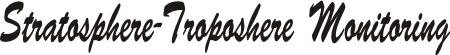The NCEP GDAS and CPC temperature and height analyses are used to monitor processes in the Stratosphere and Troposphere. In the table below are zonal mean time series of Temperature, Zonal Wind Component, Normalized Geopotential Height anomalies, amplitude of the height field's Wave 1, Wave 2, and Wave 3.

NOTE: The images for AMJ, JAS, and OND will be available at the start if their respective seasons.

2023 GDAS and CPC Zonal Mean Time Series
- T mean T anom U mean U anom Z anom Wave 1 Wave 2 Wave 3
- NH EQ SH NH EQ SH NH EQ SH NH EQ SH NH SH NH SH NH SH NH SH
Annual N E S N E S N E S N E S N S N S N S N S
JFM N E S N E S N E S N E S N S N S N S N S
AMJ N E S N E S N E S N E S N S N S N S N S
JAS N E S N E S N E S N E S N S N S N S N S
OND N E S N E S N E S N E S N S N S N S N S

Past Year's Annual Time Series
- T mean T anom U mean U anom Z anom Wave 1 Wave 2 Wave 3
- NH EQ SH NH EQ SH NH EQ SH NH EQ SH NH SH NH SH NH SH NH SH
2022 N E S N E S N E S N E S N S N S N S N S
2021 N E S N E S N E S N E S N S N S N S N S
2020 N E S N E S N E S N E S N S N S N S N S
2019 N E S N E S N E S N E S N S N S N S N S
2018 N E S N E S N E S N E S N S N S N S N S
2017 N E S N E S N E S N E S N S N S N S N S
2016 N E S N E S N E S N E S N S N S N S N S
2015 N E S N E S N E S N E S N S N S N S N S
2014 N E S N E S N E S N E S N S N S N S N S
2013 N E S N E S N E S N E S N S N S N S N S
2012 N E S N E S N E S N E S N S N S N S N S
2011 N E S N E S N E S N E S N S N S N S N S
2010 N E S N E S N E S N E S N S N S N S N S
2009 N E S N E S N E S N E S N S N S N S N S
2008 N E S N E S N E S N E S N S N S N S N S
2007 N E S N E S N E S N E S N S N S N S N S
2006 N E S N E S N E S N E S N S N S N S N S
2005 N E S N E S N E S N E S N S N S N S N S
2004 N E S N E S N E S N E S N S N S N S N S
2003 N E S N E S N E S N E S N S N S N S N S
2002 N E S N E S N E S N E S N S N S N S N S
2001 N E S N E S N E S N E S N S N S N S N S
2000 N E S N E S N E S N E S N S N S N S N S
1999 N E S N E S N E S N E S N S N S N S N S
1998 N E S N E S N E S N E S N S N S N S N S
1997 N E S N E S N E S N E S N S N S N S N S
1996 N E S N E S N E S N E S N S N S N S N S
1995 N E S N E S N E S N E S N S N S N S N S
1994 N E S N E S N E S N E S N S N S N S N S
1993 N E S N E S N E S N E S N S N S N S N S
1992 N E S N E S N E S N E S N S N S N S N S
1991 N E S N E S N E S N E S N S N S N S N S
1990 N E S N E S N E S N E S N S N S N S N S
1989 N E S N E S N E S N E S N S N S N S N S
1988 N E S N E S N E S N E S N S N S N S N S
1987 N E S N E S N E S N E S N S N S N S N S
1986 N E S N E S N E S N E S N S N S N S N S
1985 N E S N E S N E S N E S N S N S N S N S
1984 N E S N E S N E S N E S N S N S N S N S
1983 N E S N E S N E S N E S N S N S N S N S
1982 N E S N E S N E S N E S N S N S N S N S
1981 N E S N E S N E S N E S N S N S N S N S
1980 N E S N E S N E S N E S N S N S N S N S
1979 N E S N E S N E S N E S N S N S N S N S
 NOAA/ National Weather Service NOAA Center for Weather and Climate Prediction Climate Prediction Center 5830 University Research Court College Park, Maryland 20740 Page Author: Climate Prediction Center Internet Team Disclaimer Information Quality Credits Glossary Privacy Policy Freedom of Information Act (FOIA) About Us Career Opportunities